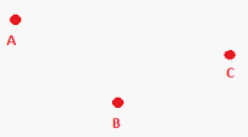# How do we represent a point in geometry?

Solution:

A point is the most fundamental object or entity in geometry. It is represented by a dot and denoted by a capital letter. A point represents position only; it has zero size (that is, zero length, zero width, and zero height).An example of a point would be an intersection of two lines. Two lines intersect in a point.An image of three points A, B, and C.

A star in the sky can be thought of a twinkling point. Similarly some other examples of points are: the tip of a compass, the sharpened end of a pencil, the pointed end of a needle.

Updated on: 10-Oct-2022

87 Views